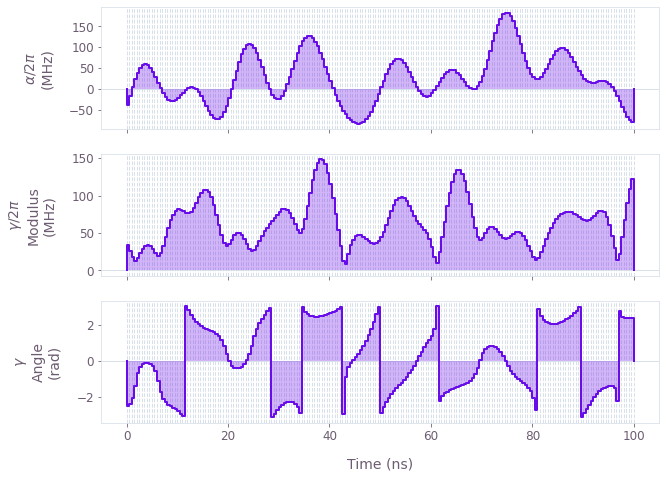•Boulder Opal
• User guides
• Optimize controls using a Fourier basis

# How to optimize controls using a Fourier basis

Create optimized pulses using CRAB techniques

Boulder Opal exposes a highly-flexible optimization engine for general-purpose gradient-based optimization. In chopped random basis (CRAB) optimization, pulses are defined via optimizable linear combinations from a set of basis functions, which can greatly reduce the dimensionality of the optimization search space. Traditionally, a randomized Fourier basis is used, although the same technique has also seen success with other bases, for example Slepian functions. You can also read the related user guides showing how to find optimal pulses using a Hann series basis or an arbitrary (user-defined) basis.

## Summary workflow

### 1. Define basis function for signal composition in the graph

The Boulder Opal optimization engine provides a convenience graph operation, graph.real_fourier_pwc_signal, for creating optimizable signals in a Fourier basis, suitable for use in a CRAB optimization. Other bases are supported by the framework, but require the user to manually provide operations that compute the appropriate linear combinations, as shown in the How to perform model-based optimization with user-defined basis functions guide.

### 2. Execute graph-based optimization

With the graph object created, an optimization can be run using the qctrl.functions.calculate_optimization function. The cost, the outputs, and the graph must be provided. The function returns the results of the optimization.

## Example: CRAB optimization on a qutrit

In this example, we perform a CRAB optimization (in the Fourier basis) of a qutrit system in which we effect a single-qubit gate while minimizing leakage out of the computational subspace. The system is described by the following Hamiltonian:

\begin{align} H(t) = & \frac{\chi}{2} (a^\dagger)^2 a^2 + (1+\beta(t)) \left(\gamma(t) a + \gamma^*(t) a^\dagger \right) + \frac{\alpha(t)}{2} a^\dagger a, \end{align}

where $\chi$ is the anharmonicity, $\gamma(t)$ and $\alpha(t)$ are, respectively, complex and real time-dependent pulses, $\beta$ is a small, slowly-varying stochastic amplitude noise process, and $a = |0 \rangle \langle 1 | + \sqrt{2} |1 \rangle \langle 2 |$.

import numpy as np
from qctrlvisualizer import plot_controls

from qctrl import Qctrl

# Start a Boulder Opal session
qctrl = Qctrl()

First, define the operators and parameters for the optimization:

# Define target and projector matrices
[[1.0, 1.0, 0], [1.0, -1.0, 0], [0, 0, np.sqrt(2)]], dtype=complex
) / np.sqrt(2)
qubit_projector = np.pad(np.eye(2), ((0, 1), (0, 1)), mode="constant")

# Define physical constraints
transmon_levels = 3
chi = 2 * np.pi * -300.0 * 1e6  # Hz
gamma_max = 2 * np.pi * 30e6  # Hz
alpha_max = 2 * np.pi * 30e6  # Hz
segment_count = 200
duration = 100e-9  # s

Create and execute the optimization graph:

# Create graph object
graph = qctrl.create_graph()

# Define standard matrices
a = graph.annihilation_operator(transmon_levels)

# Create gamma(t) signal in Fourier bases. To demonstrate the full
# flexibility, we show how to use both randomized and optimizable
# basis elements. Elements with fixed frequencies may be chosen too.
gamma_i = graph.real_fourier_pwc_signal(
duration=duration, segment_count=segment_count, randomized_frequency_count=10
)
gamma_q = graph.real_fourier_pwc_signal(
duration=duration, segment_count=segment_count, optimizable_frequency_count=10
)
gamma = graph.complex_value(gamma_i * gamma_max, gamma_q * gamma_max, name="gamma")

# Create alpha(t) signal
alpha = graph.real_fourier_pwc_signal(
duration=duration,
segment_count=segment_count,
initial_coefficient_lower_bound=-alpha_max,
initial_coefficient_upper_bound=alpha_max,
optimizable_frequency_count=10,
name="alpha",
)

# Create anharmonicity term
anharmonicity = ad2a2 * chi / 2

# Create drive term
drive = graph.hermitian_part(2 * gamma * a)

# Create clock shift term
shift = alpha * ada / 2

# Create target operator in the qubit subspace
target_operator = graph.target(
)

# Create infidelity
infidelity = graph.infidelity_pwc(
hamiltonian=anharmonicity + drive + shift,
target=target_operator,
noise_operators=[drive],
name="infidelity",
)

# Run the optimization
optimization_result = qctrl.functions.calculate_optimization(
cost_node_name="infidelity",
output_node_names=["alpha", "gamma"],
graph=graph,
optimization_count=4,
)

print(f"\nOptimized cost:\t{optimization_result.cost:.3e}")

# Plot the optimized controls
plot_controls(
{
"$\\alpha$": optimization_result.output["alpha"],
"$\\gamma$": optimization_result.output["gamma"],
}
)
Your task calculate_optimization (action_id="1599301") has started.

Optimized cost:	4.958e-08This notebook was run using the following package versions. It should also be compatible with newer versions of the Q-CTRL Python package.

PackageVersion
Python3.10.8
matplotlib3.6.3
numpy1.24.1
scipy1.10.0
qctrl20.1.1
qctrl-commons17.7.0
boulder-opal-toolkits2.0.0-beta.3
qctrl-visualizer4.4.0